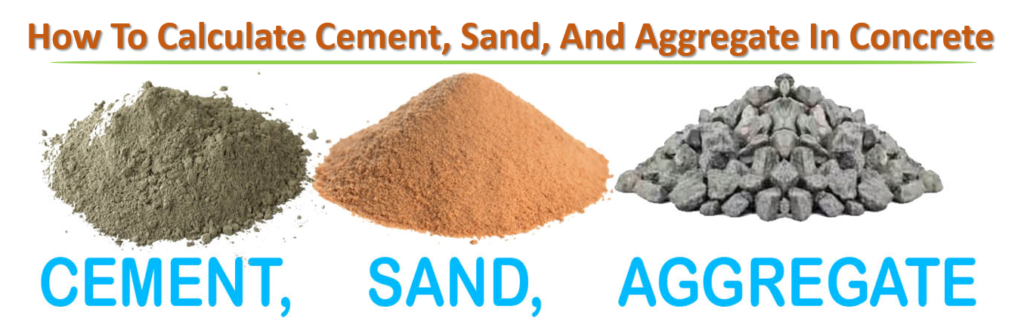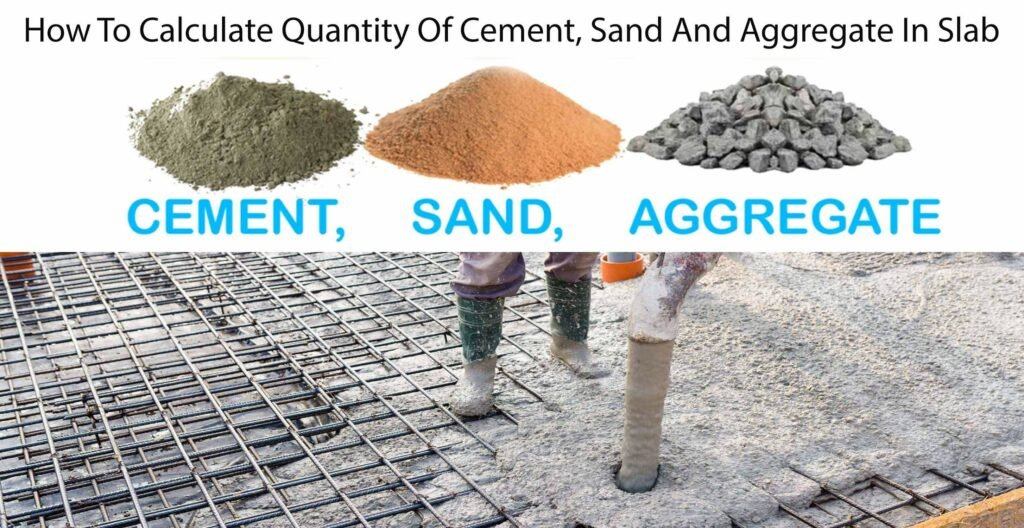Breaking News
Home / Civil Engineering / Concrete Work / How to Calculate Quantities Cement, Sand, Aggregate and water in 1 m3 Concrete Work

# How to Calculate Quantities Cement, Sand, Aggregate and water in 1 m3 Concrete Work

Well, this is the fundamentals things of Building Estimation that every civil Engineer should go through it. Concrete comprises cement, fine aggregates, coarse aggregates, and water in mix proportion.

Let us calculate the quantities

Note:  for Concrete work: Dry volume =wet volume x 1.54

For mortar work    : Dry Volume = wet volume x 1.33

Consider,

Where 1= part of cement, 2 = part of sand, 4 = parts of aggregates

Total ratio = 1+2+4 =7

Volume of concrete =1m3 (wet volume)

Dry volume = 1x 1.54 m3 = 1.54 m3

Let us know with the help of the above information I will calculate the quantities of cement, sand, and aggregates.## 1.Cement Quantity

Quantity of cement in m3 = Dry Volume x (part of cement / total ratio)

= 1.54 x (1/7) =0. 22 m3

Quantities of cement in kg = 1.54 x (1/7) x1440 = 316.8 kg (Density of cement =1440 kg/ m3)

Quantities of cement in bags = (316.8/ 50) =6.336 bags (1 bags = 50 kg)

## 2..Sand /Fine Aggregates Quantity

Quantity of sand in m3 =1.54 x (2/7) =0.44 m3

Quantity of sand in kg =( 0.44 x 1450) =638 kg (Density of sand =1450-1500)

Quantity of sand in ft3 = 0.44 x 35.3147 = 15.54 ft3 (1 m3 = 35.3147 ft3)

## 3.Coarse Aggregates Quantity

Quantity of aggregates in m3 =1.54 x (4/7) =0.88 m3

Quantity of aggregates in kg = 0.88×1500 =1320 kg (Density of aggregates =1450 -1550)

Quantity of aggregates in ft3 =0.88 x 35.3147 = 31.076 ft3

## 4.Water Quantity

Quantity of water in liter depends upon the water-cement ration and quantity of cement.

In the above examples, I have calculated

Weight of cement in m3 = 0.22 m3

Consider water-cement ration = 0.45

Amount of water required in 1 m3 concrete work = Water cement ratio x cement weight

Now, Water = 0.45 x0.220 =0.0999 m3

Amount of water required in liter= 0.0999 x 1000 = 99.9 liters.

Therefore,   For 1 m3 concrete work, 6.336 bags, 15.54 ft3 sand, 31.076 ft3

And 99.9 liters required having 1:2:4 proportions.

Let us take the example slab having a length of 5 m, a width of 3 m, and a thickness of 150 mm.

Length of slab = 5 m

Width of slab = 3 m

Thickness of slab =0.15 m

And, M15 =1:2:4 where 1 parts cement, 2 part sand, 4 part aggregates

Volume of slab = length x width x thickness

= 5 x 3 x 0.15 = 2.25 m3

We got Wet Volume = 2.25 m3

Therefore, Dry Volume =2.25 x1.54 = 3.465 m3

## Quantities calculation of Slab### 1. Cement Calculation

Volume of cement in m3 = 3.465 x (1/7)=0.495 m3

Volume of cement in bags = (0.495 x 1440) /50 = 14.25 bags

### 2.Sand calculation

Volume of sand in m3   = 3.465 x(2/7) = 0.99 m3

### 3.Aggregate calculation

Volume of aggregate in m3 = 3.465 x(4/7) =1.98 m3

### 4.Water Quantity

Quantity of water in m3 = w/c x volume of cement in m3

= 0.45 x 0.495 = 0.22275 m3

Quantity of water in liter = 0.22275 x 1000 =222.75 liters ( 1 m3 = 1000 liters)

Therefore for 2.25 m3 of slab, 14.25 bags, 0.99 m3 sand, 1.98 m3 coarse aggregates and 222.75 liters are required.

### THANKS.